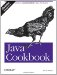# Recipe 3.4 Processing a String One Character at a Time

#### Problem

You want to process the contents of a string, one character at a time.

#### Solution

Use a for loop and the String's charAt( ) method.

#### Discussion

A string's charAt( ) method retrieves a given character by index number (starting at zero) from within the String object. To process all the characters in a String, one after another, use a for loop ranging from zero to String.length( )-1 . Here we process all the characters in a String:

`// StrCharAt.java String a = "A quick bronze fox leapt a lazy bovine"; for (int i=0; i < a.length( ); i++)     System.out.println("Char " + i + " is " + a.charAt(i));`

A checksum is a numeric quantity representing and confirming the contents of a file. If you transmit the checksum of a file separately from the contents, a recipient can checksum the file assuming the algorithm is known and verify that the file was received intact. Example 3-3 shows the simplest possible checksum, computed just by adding the numeric values of each character. Note that on files, it does not include the values of the newline characters; to fix this, retrieve System.getProperty("line.separator"); and add its character value(s) into the sum at the end of each line. Or give up on line mode and read the file a character at a time.

##### Example 3-3. CheckSum.java
`/** CheckSum one file, given an open BufferedReader. */     public int process(BufferedReader is) {         int sum = 0;         try {             String inputLine;             while ((inputLine = is.readLine( )) != null) {                 int i;                 for (i=0; i<inputLine.length( ); i++) {                     sum += inputLine.charAt(i);                 }             }             is.close( );         } catch (IOException e) {             System.out.println("IOException: " + e);     } f     return sum;  }`Java Cookbook, Second Edition
ISBN: 0596007019
EAN: 2147483647
Year: 2003
Pages: 409
Authors: Ian F Darwin

Similar book on Amazon